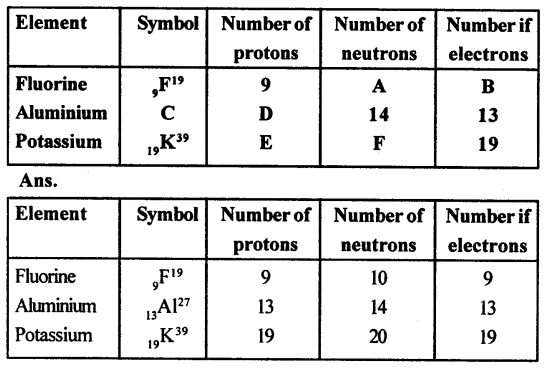## Selina Concise Chemistry Class 8 ICSE Solutions – Atomic Structure

ICSESolutions.com provides step by step solutions for Selina Concise ICSE Solutions for Class 8 Chemistry Chapter 4 Atomic Structure. You can download the Selina Concise Chemistry ICSE Solutions for Class 8 with Free PDF download option. Selina Publishers Concise Chemistry for Class 8 ICSE Solutions all questions are solved and explained by expert teachers as per ICSE board guidelines.

Exercise

1. Fill in the blanks.

(a) Dalton said that atoms could not be divided
(b) An ion which has a positive charge is called a cation.
(c) The outermost shell of an atom is known as valence shell.
(d) The nucleus of an atom is very hard and dense.
(e) Neutrons are neutral particles having mass equal to that of protons.
(f) Isotopes are the atoms of an element having the same atomic number but a different mass number.

2. Write ‘true’ or ‘false’ for the following statements:

(a) An atom on the whole has a positive charge.
false
(b) The maximum number of electrons in the first shell can be 8.
false
(c) The central pad of the atom is called nucleus.
True.

3. Give the following a suitable word/phrase.

(a) The sub-atomic particle with negative charge and negligible mass.
(b) Protons and neutrons present in the nucleus.
(c) The electrons present in the outermost shell.
(d) Arrangement of electrons in the shells of an atom.
(e) The number of protons present in the nucleus of an atom.
(f) The sum of the number of protons and neutrons of an atom.
(g) Atoms of same element with same atomic number but a different mass number.
(h) The smallest unit of an element which takes part in a chemical reaction.

(a) Neutron
(b) Mass number
(c) Valency
(d) Orbits or Valence shells
(e) Atomic number
(f) Mass number
(g) Isotopes
(h) Atom

4. Multiple Choice Questions

(a) The outermost shell of an atom is known as

1. valency
2. valence electrons
3. nucleus
4. valence shell

(b) The number of valence electrons present in magnesium is

1. two
2. three
3. four
4. five

(c) The sub atomic particle with negative charge is

1. proton
2. neutron
3. electron
4. nucleon

(d) If the atomic number of an atom is 17 and mass number is 35 then number of neutron will be

1. 35
2. 17
3. 18
4. 52

(e) The number of electrons in an atom is equal to number of

1. protons in a neutral atom
2. neutrons in a neutral atom
3. nucleons in a neutral atom
4. none of the above

(f) The sum of number of protons and number of neutrons present in the nucleus of an atom is called its

1. mass number
2. atomic number
3. number of electrons
4. all of the above

Question 5.
Name three fundamental particles of the atom. Give the symbol with charge, on each particle.
The fundamental particles of the atom are: electrons, protons and neutrons.

 Particle Symbol Charge electron e– -1 or 1.602 x 10-19 C. Where -1 represent its one unit negative electrical charge proton p+ + 1 or 1.602 x 10-19 C. Where +1 represents one unit +ve electrical charge. neutron no 0

Question 6.
Define the following terms:
(a) Atomic number
(b) Mass number
(c) Nucleons
(d) Valence shell
(a) Atomic number: Atomic number refers to the number of protons present in an atom. It is denoted by Z. Example: An atom of oxygen contains 8 proton Therefore its atomic number is 8.
(b) Mass number: Mass number refers to the sum of the number of protons and neutrons present in the nucleus of an atom and denoted by A Mass number = Number of protons + Number of neutrons.
(c) Nucleons: The protons and neutrons collectively are known as nucleons.
(d) Valence Shell: The outermost shell of an atom is known as its valence shell.

Question 7.
Mention briefly the salient features of Dalton’s atomic theory (five points).
Salient features of Dalton’s atomic theory:

1. Matter consists of very small and indivisible particles called atoms, which can neither be created nor can be destroyed.
2. The atoms of an element are alike in all respects i.e. size, mass, density, chemical properties but they differ from the atoms of other elements.
3. Atoms of an element combine in small numbers to form molecules of the element.
4. Atoms of one element combine with atoms of another element in simple whole number ratio to form molecules of compounds.
5. Atoms are the smallest units of matter that can take part in a chemical reaction during which only rearrangement of atoms takes place.

Question 8.
(a) What are the two main features of Rutherford’s atomic model?
(b) State its one drawback.
(a) According to Rutherford’s model an atom consists of:

1. The centrally located nucleus: The nucleus is a centrally located positively charged mass. The entire mass of the atom is concentrated in it. It is the densest part of the atom. Its size is very small as compare to the atom as a whole.
2. The outer circular orbits: Electrons revolve in circular orbits (shell) in the space available around the nucleus. An atom is electrically neutral i.e., number of protons and electrons present in an atom are equal.

(b) Rutherford’s atomic model could not explain the stability of the atom as it is like a solar system, the sun is at the centre and the planets revolve around it, in an atom the electrons revolve around the centrally located nucleus containing protons.

Question 9.
What are the observations of the experiment done by Rutherford in order to determine the structure of an atom?
Following were the observations made by Rutherford:

1. Most of the alpha particle passed straight through the foil without any deflection from their path.
2. A small fraction of them were deflected from their original path by small angles.
3. Only a few particles bounced back.

Question 10.
State the mass number, the atomic number, number of neutrons and electronic configuration of the following atoms.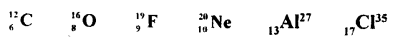Also, draw atomic diagrams for them.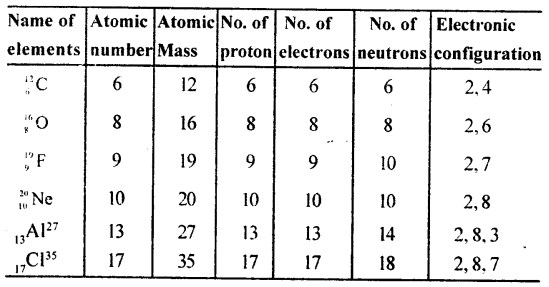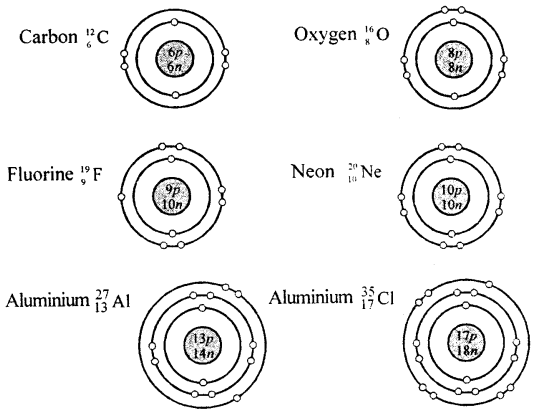Question 11.
What is variable valency? Name two elements having variable valency and state their valencies.
Variable valency: Some elements exhibit more than one valency. They are said to have variable valency, e.g. Iron, copper, tin, lead.
Iron         Fe       Fe2+ or Fe3+
Copper   cu        cu+ or cu2+

Question 12.
The atomic number and the mass number of sodium are 11 and 23 respectively. What information is conveyed by this statement?
Atomic number = 11; No of protons = 11
Mass number = 23 = Number of protons + Number of neutrons.
No of neutrons = 23-11 = 12.

Question 13.
Draw the diagrams representing the atomic structures of the following:
(a) Nitrogen (b) Neon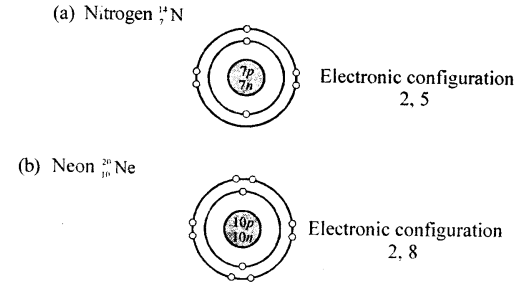Question 14.
Explain the rule with example according to which electrons are filled in various energy levels,
The maximum number of electrons that can be present in any shell or orbit of an atom is given by the formula 2n2, where n is the serial number of the shell.
Therefore:
K shell, n = 1, no. of electrons = 2 x 12 = 2
L shell, n = 2, no. of electrons = 2 x 22 = 8
M shell, n = 3, no. of electrons = 2 x 32 = 18
N shell, n = 4, no. of electrons = 2 x 42= 32
Electrons are not accommodated in a given shell, unless the inner shells are filled.
That is, the shells are filled in a stepwise manner.
Example:Question 15.
The atom of an element is made up of 4 protons, 5 neutrons and 4 electrons. What is its atomic number and mass number?
Protons = 4, neutrons = 5, electrons = 4
Atomic number = 4,
Mass number = 4 + 5 = 9

Question 16.
(a) What are the two main parts of which an atom is made of?
(b) Where is the nucleus of an atom situated ?
(c) What are orbits or shells of an atom ?
(a)

1. The centrally located nucleus
2. The outer circular orbits.

(b) The nucleus is a centrally located positively charged mass.
(c) The circular orbits (shell present) in the space available around the nucleus on which electrons revolve are called orbits or shells of an atom.

Question 17.
What are isotopes? How does the existence of isotopes contradict Dalton’s atomic theory?